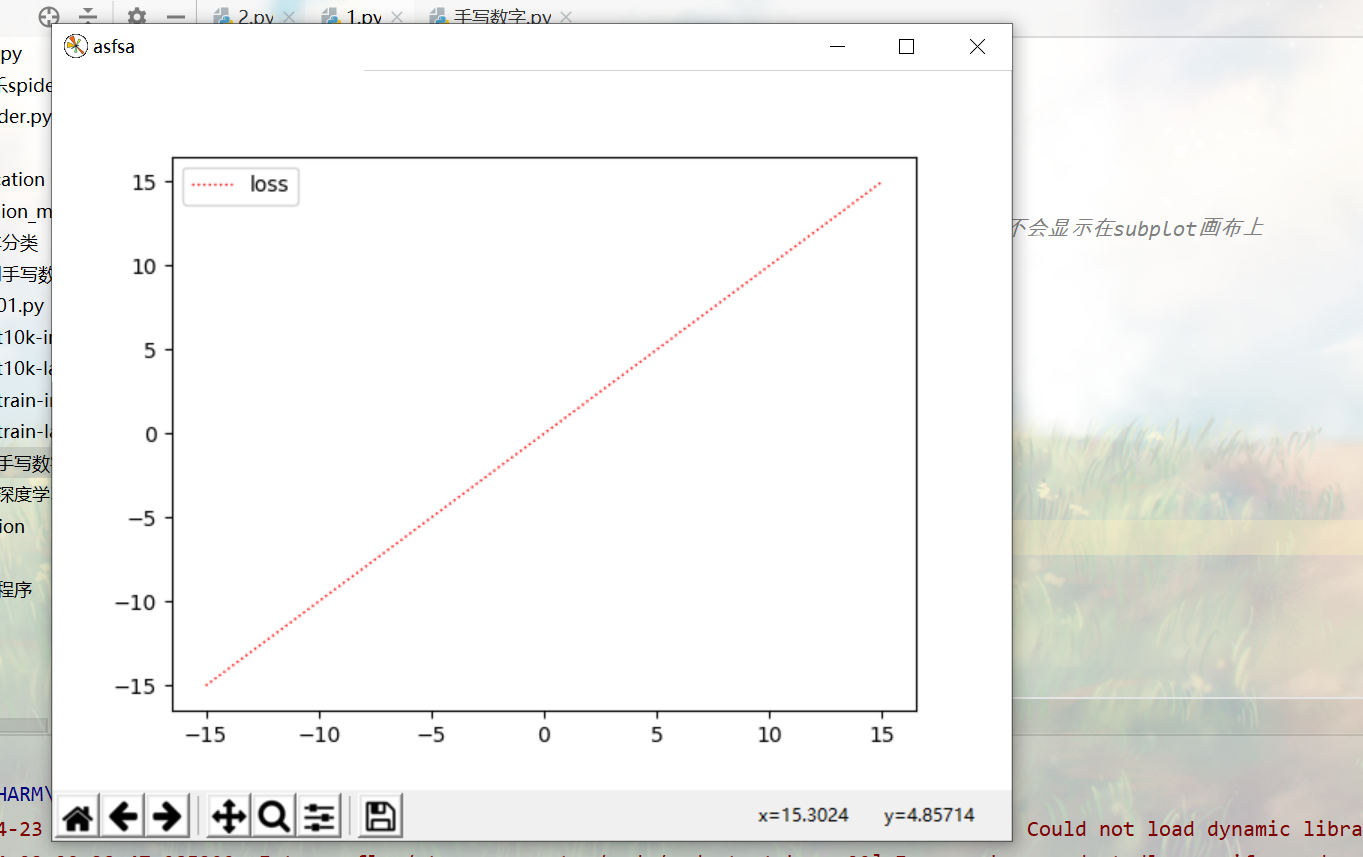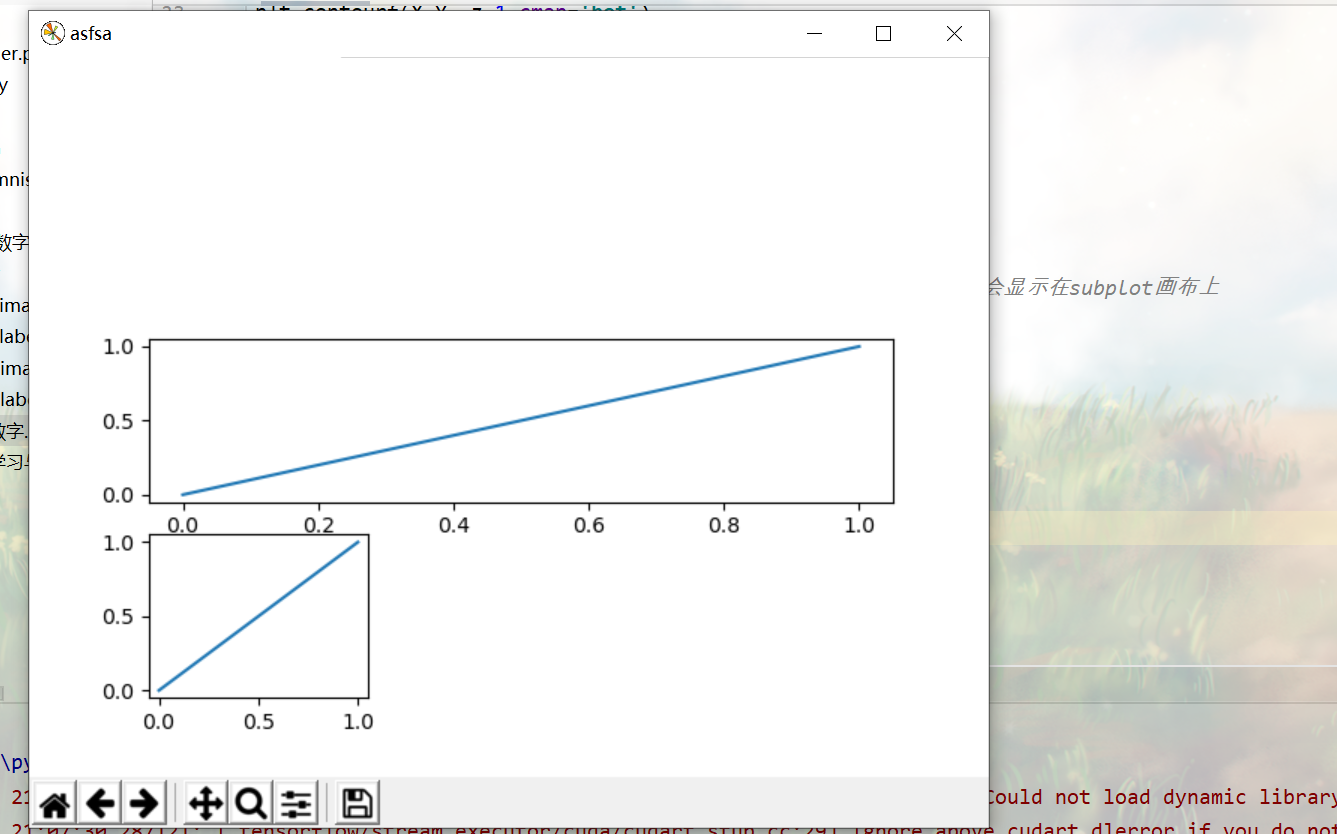matplotlib 基础|笔记

https://www.bilibili.com/video/BV1Jx411L7LU?

plt.rcParams['font.sans-serif'] = ['SimHei'] # 用来正常显示中文标签

plt.rcParams['axes.unicode_minus'] = False # 用来正常显示负号

p0:折线图

plt.plot(x,y,label)

p4：创建画布

#创建第num个画布，名称为num facecolor 是背景颜色，edgecolor 是边的颜色
plt.figure(num=1,facecolor,edgecolor)

p6：设置坐标轴|label

plt.xlim(5,15)
plt.ylim(5,15)
# 关闭坐标轴
plt.axis('off')
# 关闭坐标轴之后标签也不会显示 （鸡肋？）
# 修改x，y的标签
plt.xlabel(string)
plt.ylabel(string)

p7：Legend# 这里用直线图举例直线图
# linestyle 可以设置线的形式比如细点画线等等，linewidth是线的粗细
l1,=plt.plot(x,y,color='red',linewidth=1,linestyle=':')
l2,=plt.plot(x,y2)
#将x轴的坐标改成自己想显示的坐标  $...$ 可以变斜体

plt.xticks([10,20,30,40],[r'$asdf$',r'$asdfg$',r'qerqr']) #在图旁显示线的名称，之前要声明l1,=plt... 逗号不能省，loc=best 代表自动寻找最好的地方显示 plt.legend(handles=[l1,],labels=['loss'],loc='best')

plt.bar(x,y,label='string%.2f'% xx)
plt.legend()

a=plt.set_text(x,y,string)|text(string) 也可以设置文本(string)和文本位置 (x,y)|可省略

a.set_posititon(x,y) 还可以单独设置文本位置

p10也有实例

p10：散点图（scatter）

#s是size，alpha 是透明度 c是color |这个color设置很迷
C=np.arctan2(y1 ,x)

plt.scatter(x,y,s=3,c=C,alpha=0.6)

p11：柱状图

# 柱状图 facecolor 柱状图颜色|这个代码可以百度上找 edgecolor 边框颜色
plt.bar(x,y,facecolor='#9999ff',edgecolor='white')
plt.bar(x,-y,facecolor='#ff9999',edgecolor='white')
#取出每对x，y 在柱状图上标记text
for (x,y) in zip(x,y):
# 文本的坐标 x,y, 文字, 横向对齐方式的位置|居中，纵向对齐的方式| 顶部或底部 默认bottom
# alpha 文本是透明度
plt.text(x,y+0.04,'%.2f'%y ,ha='center',va='bottom',alpha=0.6)
plt.text(x,-y-0.04,'%.2f'%-y,ha='center',va='top')

plt.barh(x=y,y=x) 是做横向柱状图，参数都差不多，就是x和y要反过来才是你想要的结果

p12：等高线图

# 等高线：底图其实是个网格，然后根据这个网格画等高线
# 将x，y绑定成一个网格
(X,Y)=np.meshgrid(x,y)
z=X**2+Y**2+np.random.normal(0,1)
# 填充颜色，contour +fillcolor ==>contourf
# x,y,是坐标 数字代表有数字+1条线<==>数字+2个颜色，z是高度，cmap是每个高度对应在cmap中的颜色 有hot和cool两种
plt.contourf(X,Y ,z,1,cmap='hot')
# 画等高线，linewidth不知道为什么报错
line=plt.contour(x,y,z,colors='black',linewidths=0.5)
# 加label contour+label==>clabel?
# inline 代表是否在线里面写字,一般为True 更好看 fontsize 字体大小
plt.clabel(line,inline=True,fontsize=8)

p13：image 图片

plt.imshow(x)
plt.colorbar() 可以将像素的rgb值从高到低排列放到一个柱状图中

p14：3D图

from mpl_toolkits.mplot3d import Axes3D
#创建画布
fig=plt.figure()
x=np.linspace(-15,15,30)
# 创建一个空子图
ax=Axes3D(fig)
y=x
#将x，y绑定成网格 就和等高线类似
x,y=np.meshgrid(x,y)
z=x**2+y**2
# 画表面 x，y，z，cmap图的颜色，edgecolor 线条颜色，
ax.plot_surface(x,y,z,cmap=plt.get_cmap('rainbow'),edgecolor='black')
# 画投影平面
# zdir=x 是从x轴往下看==> 投影到其他两个轴围成的平面|默认是z
ax.contourf(x,y,z,zdir='z')

p15+16：subplot

在一个网格上画多次，然后合并在一起显示

# 一个画布分为几行行，几列，当前画在第几个
# 先分3*3 在第7个位置画图|相当于第三行第一个
plt.subplot(3,3,7)
plt.plot([0,1],[0,1])
#再分3*1 再第二个位置画图|相当于第二行第一个 一整块
plt.subplot(3,1,2)
plt.plot([0,1],[0,1])
plt.show()在一个画布上画一个网格，分格子显示

subplot2grid

# 网格的shape，loc 图的起点， 所占行，所占列
f1=plt.subplot2grid((3,3),(0,0),rowspan=2,colspan=2)
(X,Y)=np.meshgrid(x,y)
z=X**2+Y**2+np.random.normal(0,1)
plt.contourf(X,Y ,z,1,cmap='hot')
line=f1.contour(x,y,z,colors='black',linewidths=0.5)
plt.clabel(line,inline=True,fontsize=8)

f2=plt.subplot2grid(shape,loc,rowspan,colspan)
f2.scatter()
...
plt.show()

gridspec

from matplotlib import gridspec
gs=gridspec.GridSpec(4,4) 输入网格的行列数 （4*4）
f1=plt.subplot(gs[0,1：3]) plt.plot(x,y) plt.show()

3.subplots

figure,axs=plt.subplots(n，m)   n*m的图默认1*1
axs[i][j]来指定第几个子图画

p19：Animation

# 更新的函数，传入的是frame,如果frame是为一个列表的话，更新函数调用时传入的值会是相应索引下的元素，此时动画终点就是取完列表元素
# 如果不指定 frames 则会一直传入帧序号保持下去。
# 返回你想要图上能看到的东西 因为是元组所以逗号不能漏,也可以直接打印一遍
def update(i): line.set_data(x+i,y+i) # ax_text.set_text('string')

# 或者这样更容易理解
ax.plot(x+i,y+i,label='xxxx')
ax.legend() 　　return ax,
return line,#ax_text,
# 初始化最开始的图（似乎和line的初始化差不多？，不要也可以运行...） def init(): line.set_data(x,y)
ax.set_xlim() 这里是在子图上设置而不是line
return line, if __name__=='__main__': x=np.linspace(0,2*np.pi,100) y=x # 定义画布和子图 fig,ax=plt.subplots() # 初始化子图,在子图上画线，文本 等东西 line,=ax.plot([],[],'r') #text_ax=plt.text(x=,y=,'')
ani=FuncAnimation(fig=fig,frames=100,func=update,interval=10,blit=True) plt.show()

如果动态图有的坐标没有显示改一下blit说不定就好了...

组合尝试：

柱状图+animation

import tensorflow as tf
import numpy as np
from matplotlib import pyplot as plt
import matplotlib.gridspec as gridspec
from matplotlib.animation import FuncAnimation
from mpl_toolkits.mplot3d import Axes3D
plt.rcParams['font.sans-serif'] = ['SimHei']  # 用来正常显示中文标签
plt.rcParams['axes.unicode_minus'] = False  # 用来正常显示负号
def update(frame):
ax.cla()# 清除当前轴域
ax.barh(y,x[frame],color='#9ACD32',height=0.5)
print(y)
return ax,#ax_text,
def init():
ax.set_xlim(100,300)
return ax,
if __name__=='__main__':
x=np.random.randint(100,200,size=(6,6))
y=['湖北','河北','江西','陕西','内蒙','安徽']
x_data=[]
fig,ax=plt.subplots()
ax.barh(y,x,color='#9ACD32',height=0.5)
ani=FuncAnimation(fig=fig,frames=6,func=update,interval=1000,blit=False)
plt.show()

3D+animation

因发现了pyechart 发现了新大陆，果断抛弃了matplotlib...

https://www.sioe.cn/yingyong/yanse-rgb-16/

$道路千万条,点赞第一条;阅读不规范,笔者两行泪$
posted @ 2020-04-24 22:52  Sakura_Momoko  阅读(309)  评论(0编辑  收藏  举报